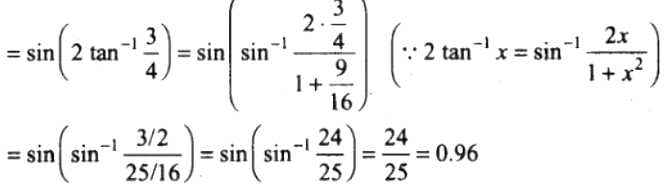# NCERT Exemplar Solutions for Class 12 Maths Chapter 2 Inverse Trigonometric Functions

Referring to subject-wise NCERT Exemplar textbooks for various classes aids students in getting thorough with the concepts covered in their class. Further, students can access NCERT Exemplar Solutions to learn the steps involved in solving the exercise problems of every chapter. All these solutions are created by subject experts following the latest CBSE pattern.

In Chapter 2 of NCERT Exemplar Solutions for Class 12 Maths is Inverse Trigonometric Functions, students will learn concepts related to inverse trigonometry functions and their properties. Moreover, they will learn the restrictions on ranges and domains of trigonometric functions. Further, they will record their behaviour through graphical representations. Students who wish to learn the right methods of solving these exercise problems can make use of the solutions PDF of NCERT Exemplar Solutions for Class 12 Maths Chapter 2 Inverse Trigonometric Functions from the link given below.

## Download the PDF of NCERT Exemplar Solutions for Class 12 Maths Chapter 2 Inverse Trigonometric Functions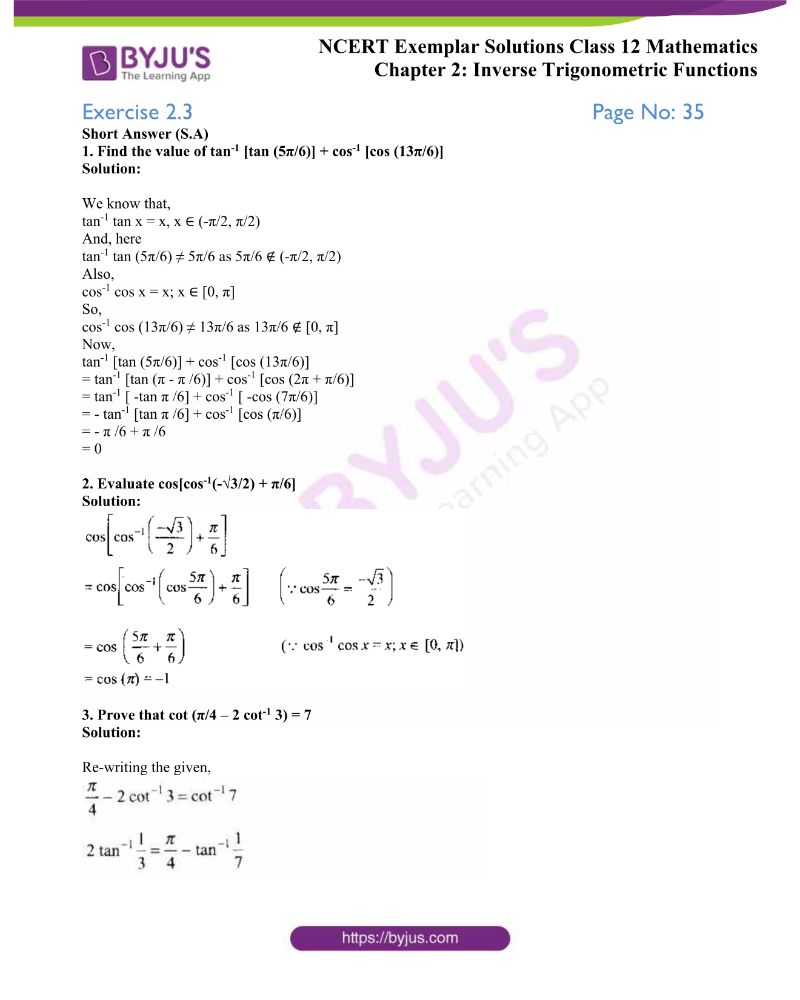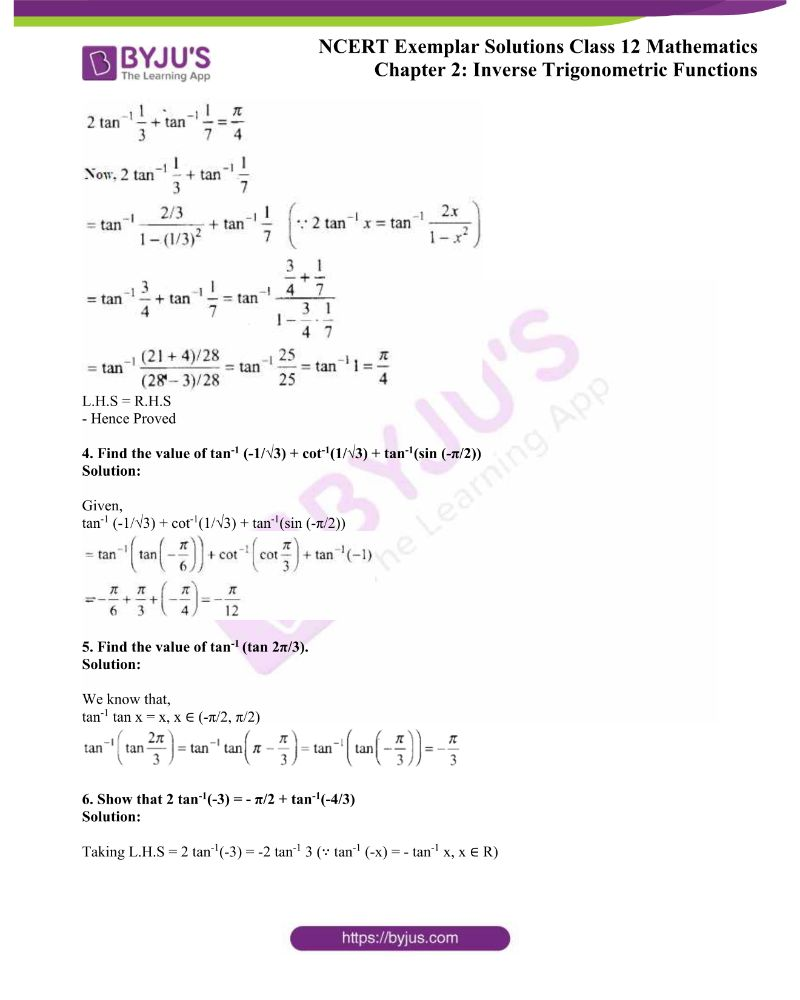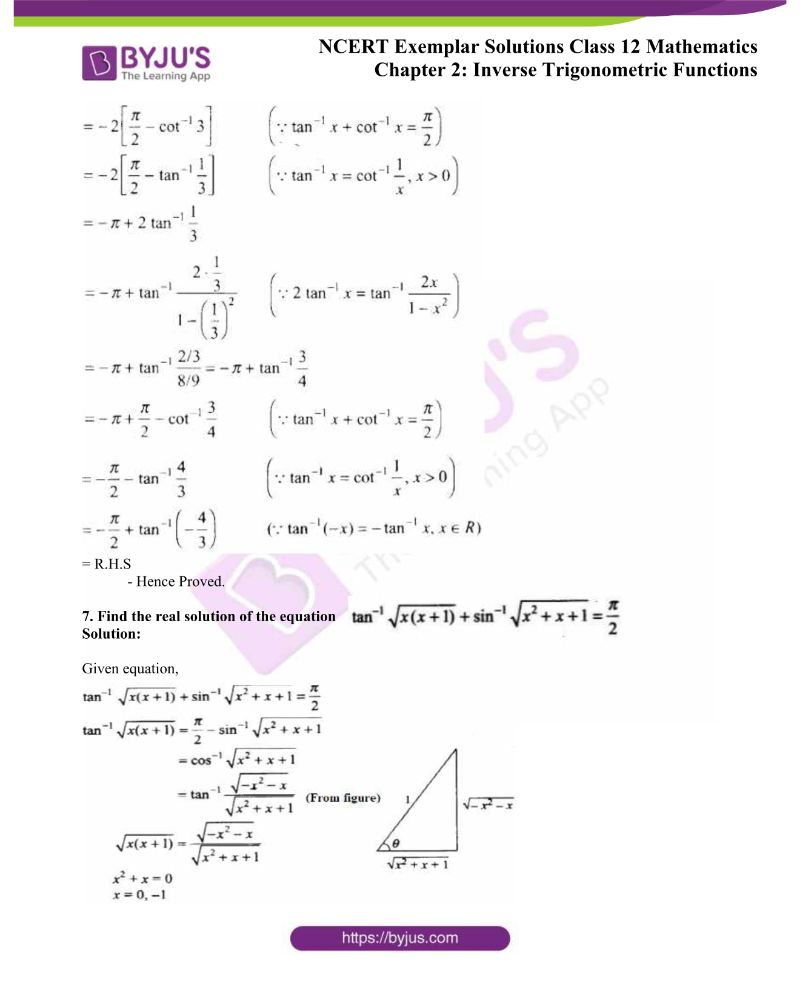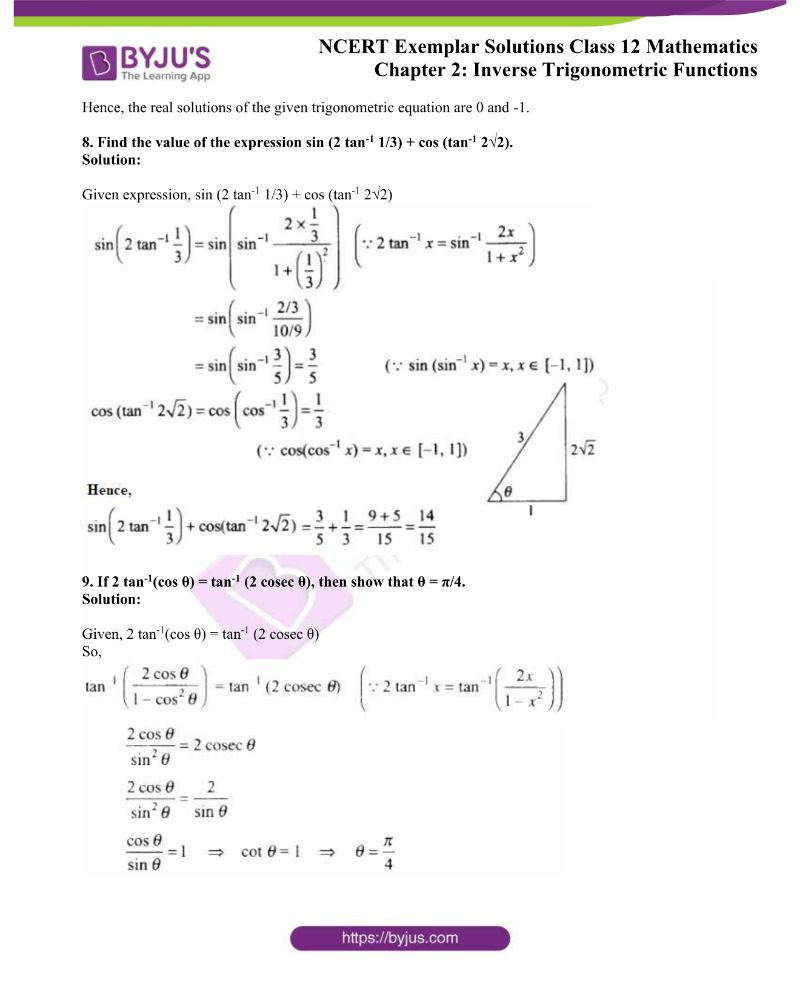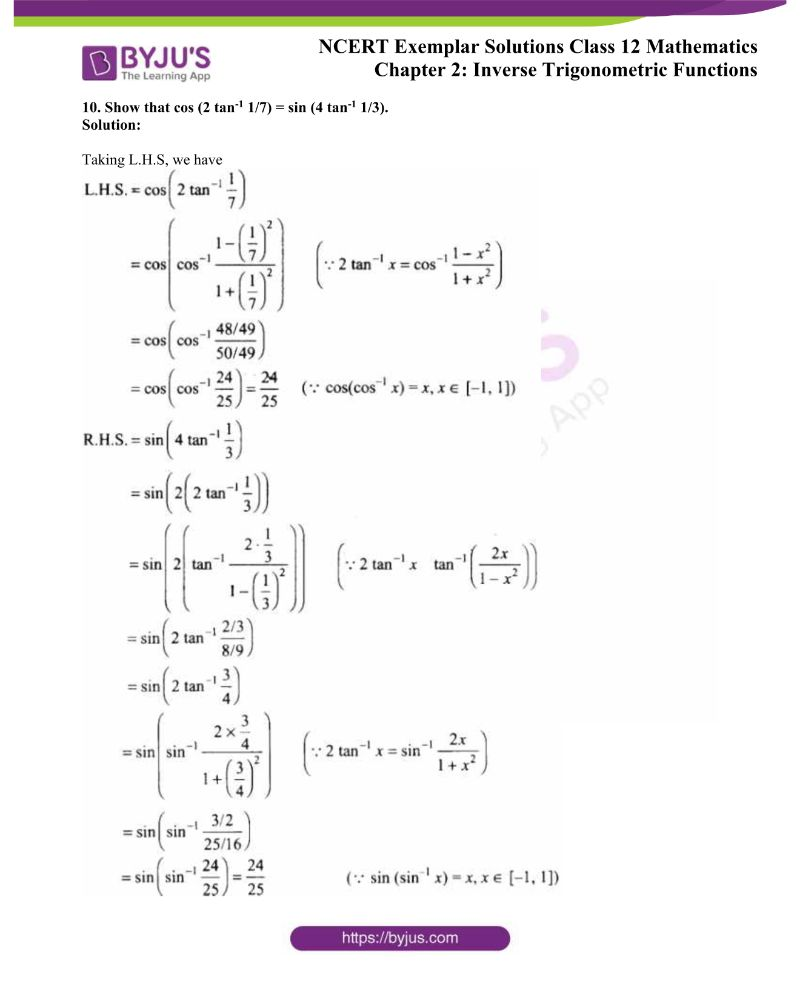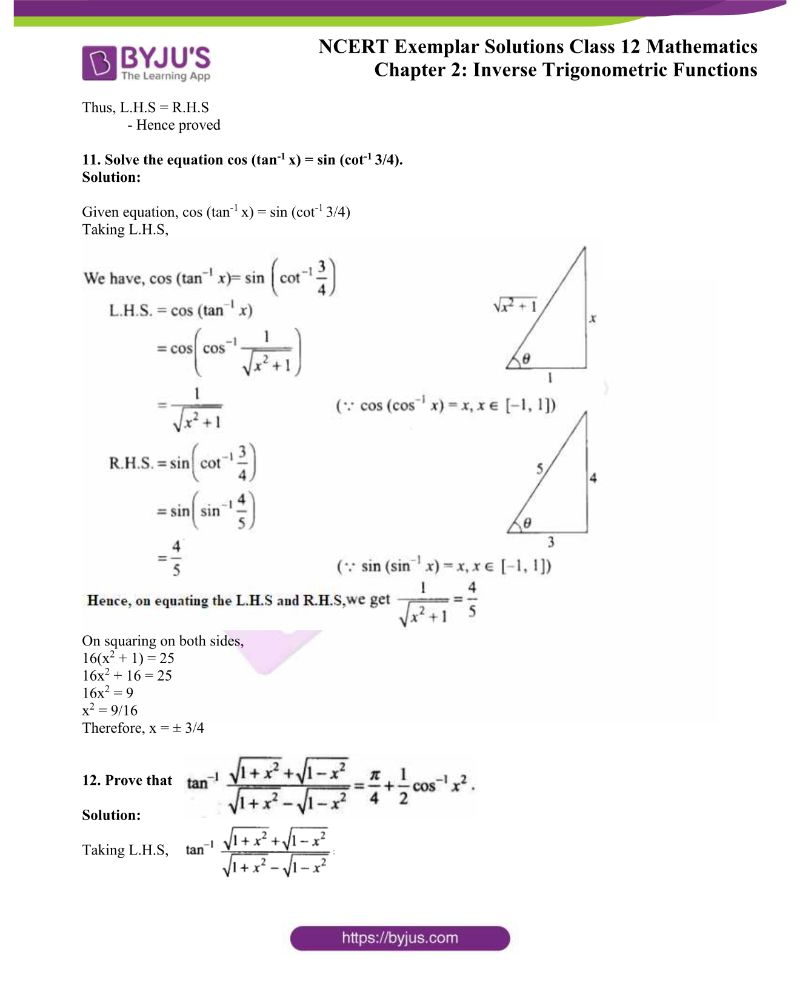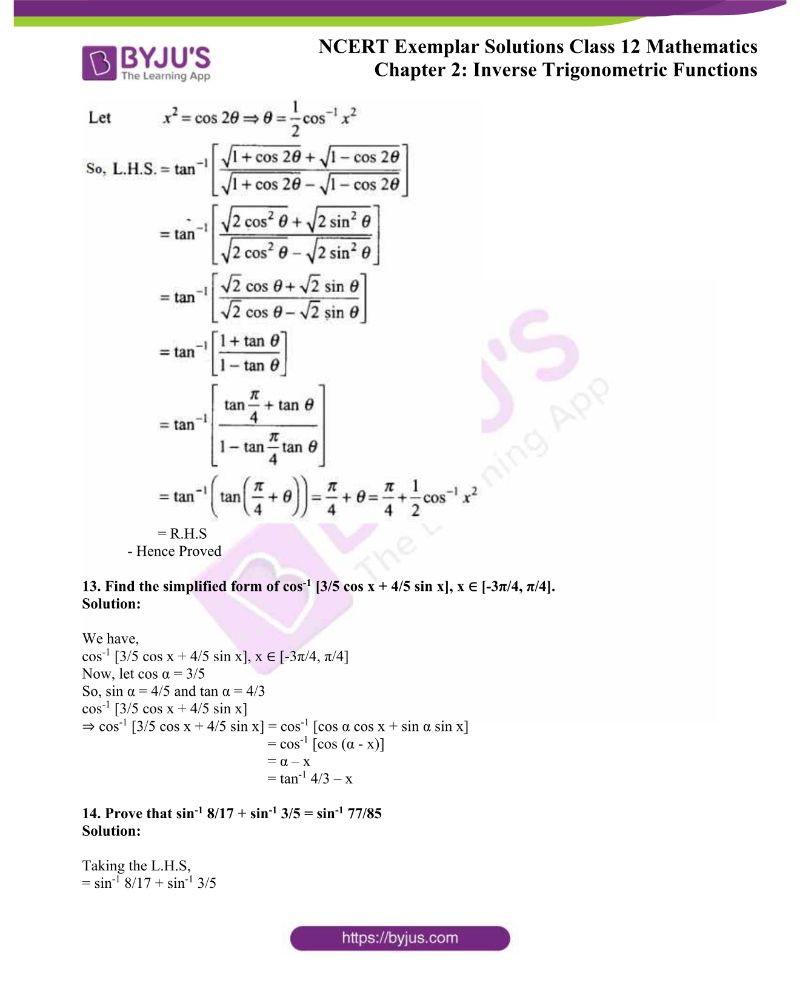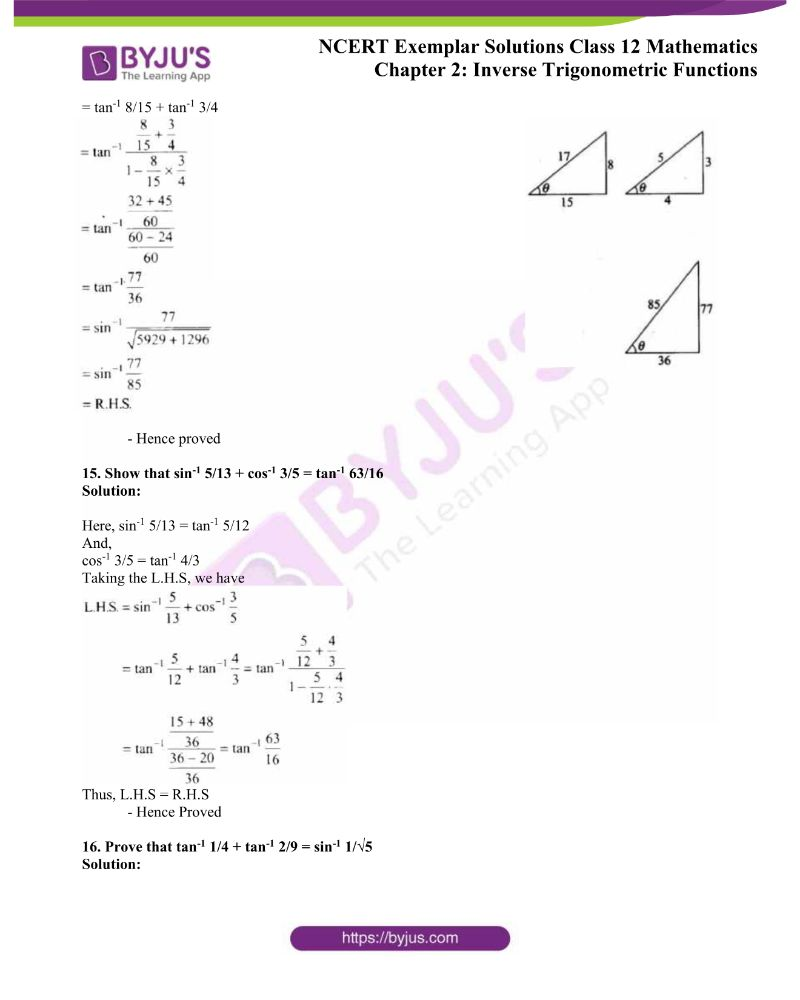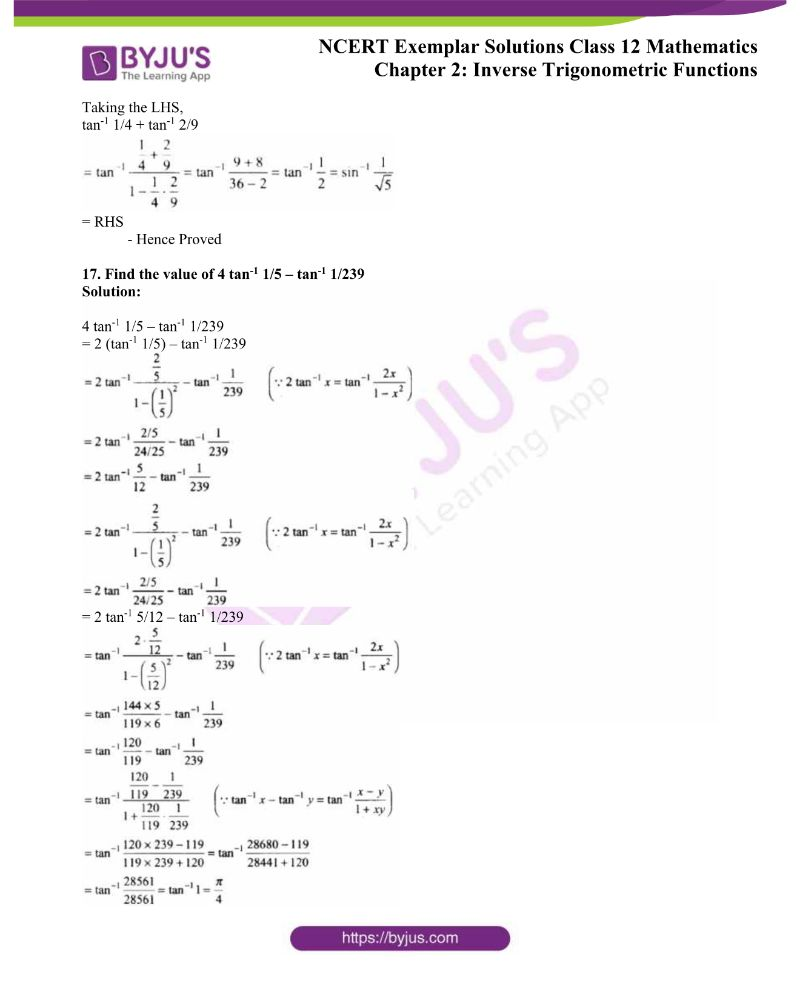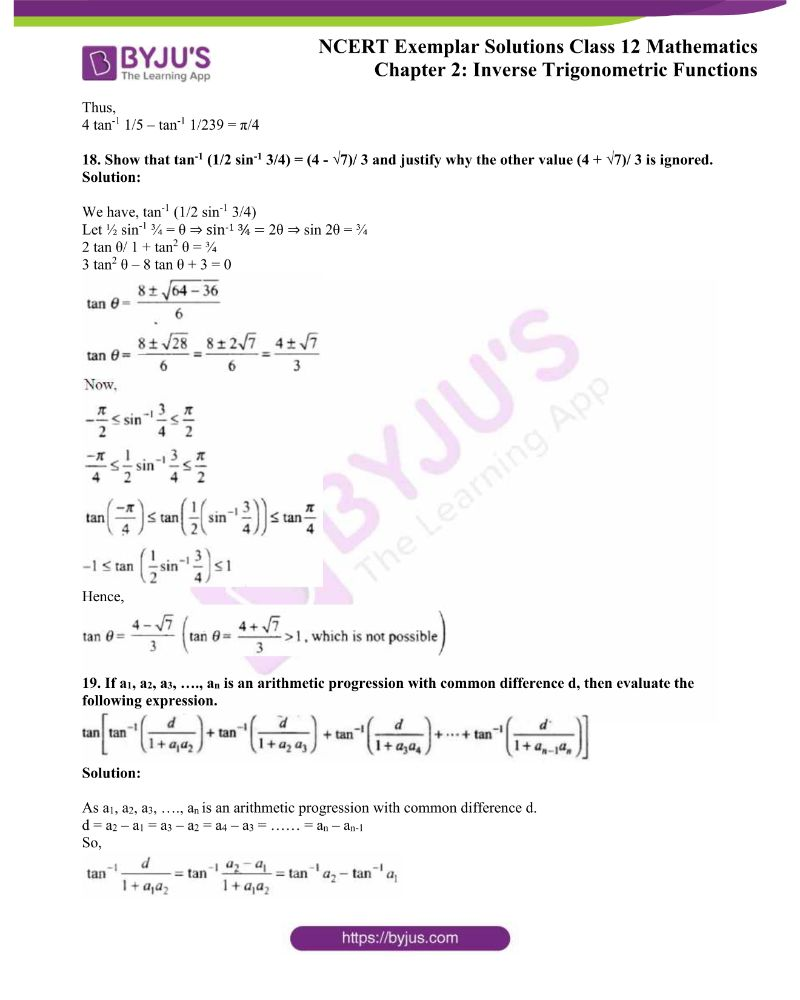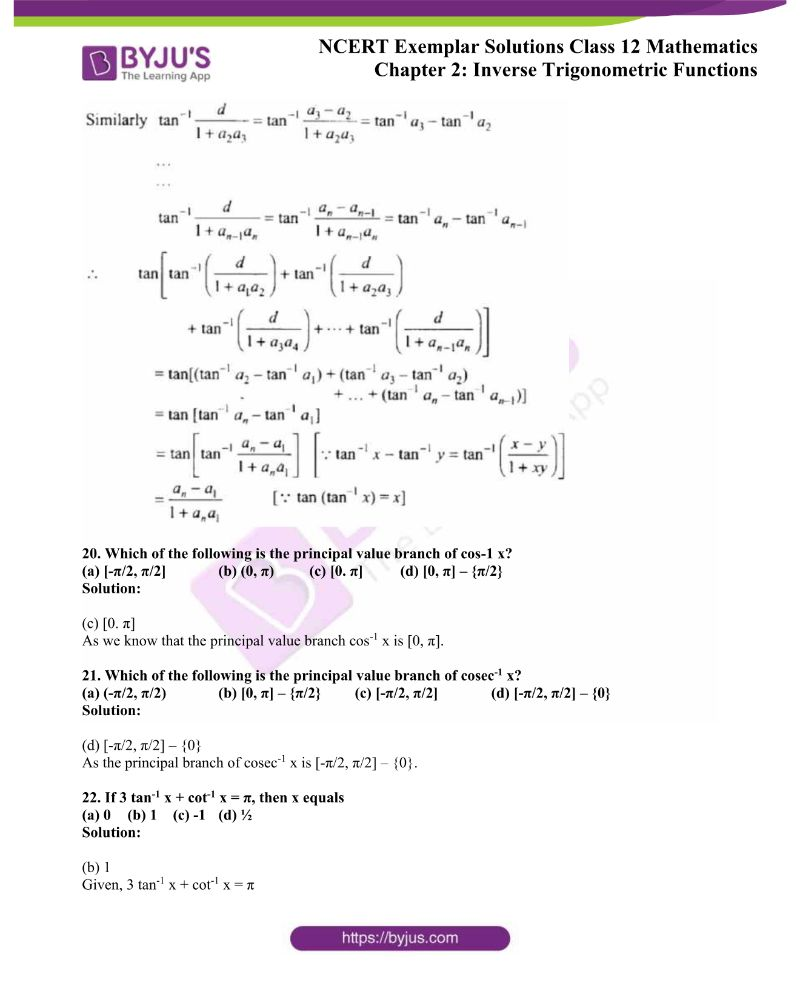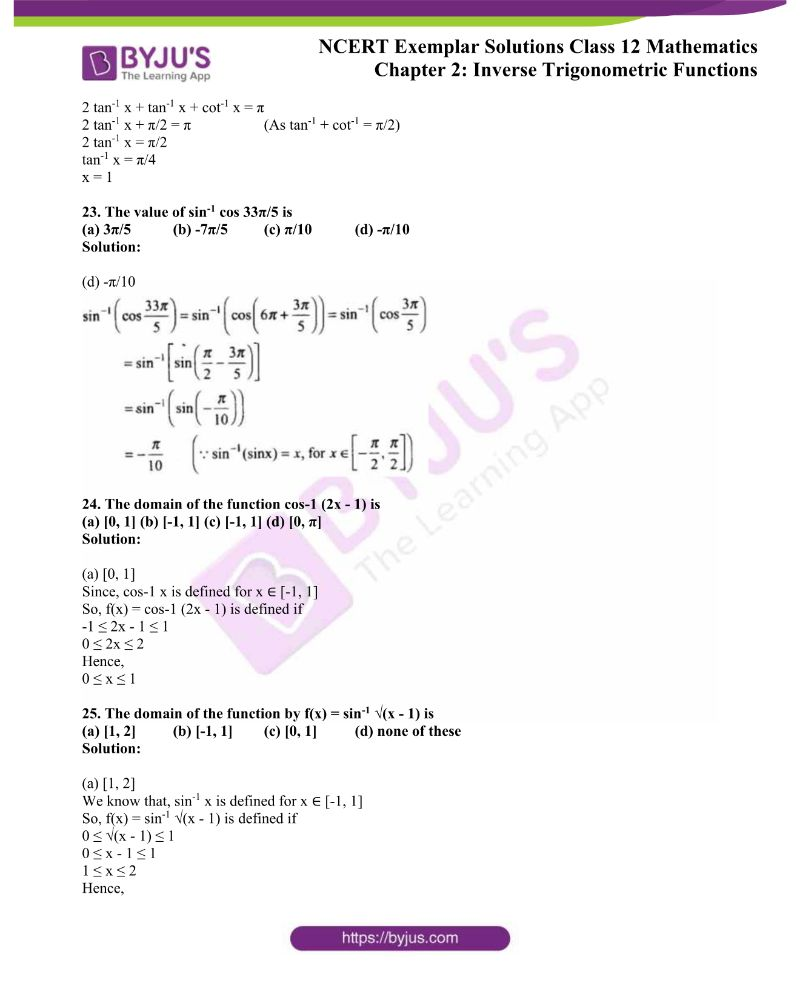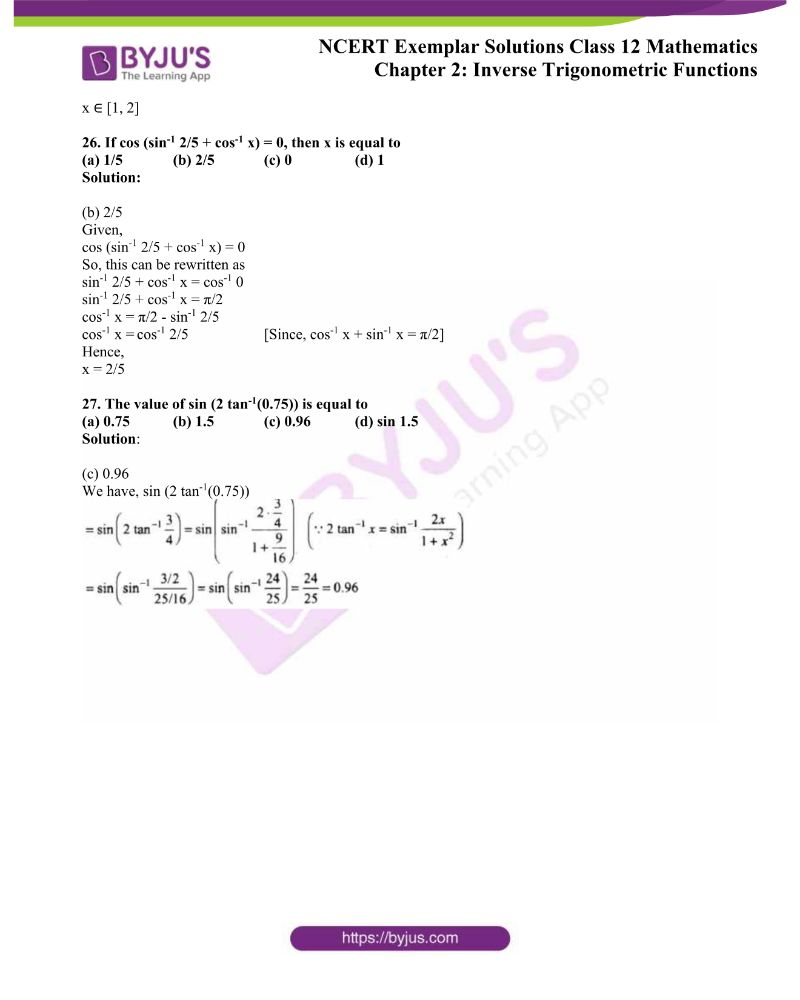### Access Answers to NCERT Exemplar Solutions for Class 12 Maths Chapter 2 Inverse Trigonometric Functions

Exercise 2.3 Page No: 35

1. Find the value of tan-1 [tan (5π/6)] + cos-1 [cos (13π/6)]

Solution:

We know that,

tan-1 tan x = x, x ∈ (-π/2, π/2)

And, here

tan-1 tan (5π/6) ≠ 5π/6 as 5π/6 ∉ (-π/2, π/2)

Also,

cos-1 cos x = x; x ∈ [0, π]

So,

cos-1 cos (13π/6) ≠ 13π/6 as 13π/6 ∉ [0, π]

Now,

tan-1 [tan (5π/6)] + cos-1 [cos (13π/6)]

= tan-1 [tan (π – π /6)] + cos-1 [cos (2π + π/6)]

= tan-1 [ -tan π /6] + cos-1 [ -cos (7π/6)]

= – tan-1 [tan π /6] + cos-1 [cos (π/6)]

= – π /6 + π /6

= 0

2. Evaluate cos[cos-1(-√3/2) + π/6]

Solution: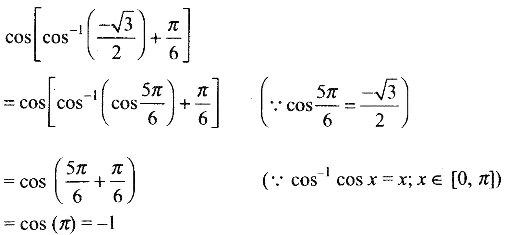3. Prove that cot (π/4 – 2 cot-1 3) = 7

Solution:

Re-writing the given,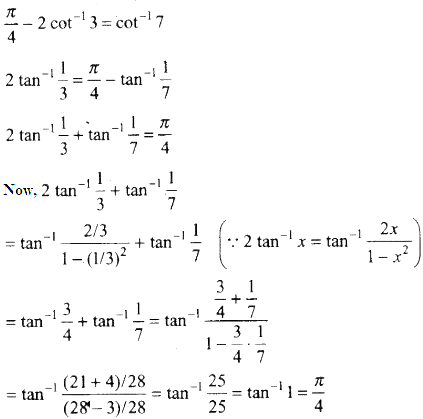L.H.S = R.H.S

– Hence Proved

4. Find the value of tan-1 (-1/√3) + cot-1(1/√3) + tan-1(sin (-π/2))

Solution:

Given,

tan-1 (-1/√3) + cot-1(1/√3) + tan-1(sin (-π/2))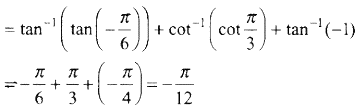5. Find the value of tan-1 (tan 2π/3).

Solution:

We know that,

tan-1 tan x = x, x ∈ (-π/2, π/2)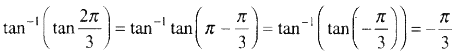6. Show that 2 tan-1(-3) = – π/2 + tan-1(-4/3)

Solution:

Taking L.H.S = 2 tan-1(-3) = -2 tan-1 3 (∵ tan-1 (-x) = – tan-1 x, x ∈ R)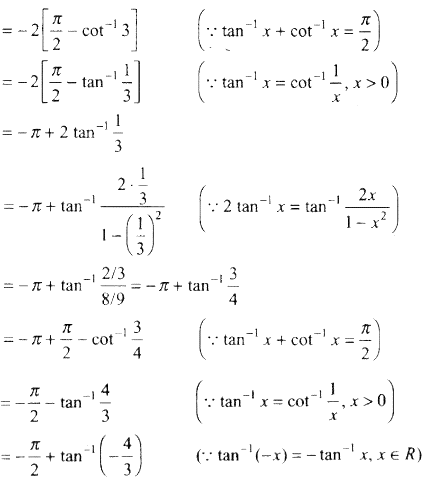= R.H.S

– Hence Proved.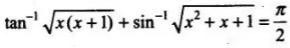7. Find the real solution of the equation

Solution:

Given equation,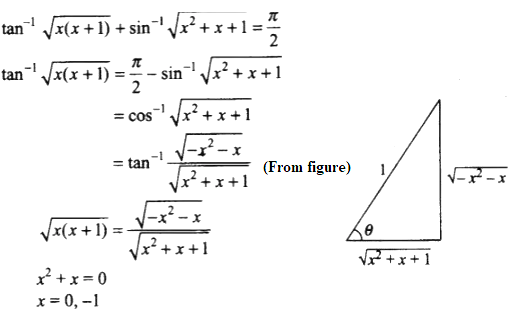Hence, the real solutions of the given trigonometric equation are 0 and -1.

8. Find the value of the expression sin (2 tan-1 1/3) + cos (tan-1 2√2).

Solution:

Given expression, sin (2 tan-1 1/3) + cos (tan-1 2√2)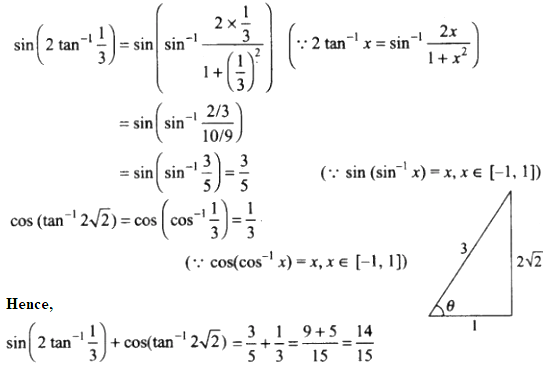9. If 2 tan-1(cos θ) = tan-1 (2 cosec θ), then show that θ = π/4.

Solution:

Given, 2 tan-1(cos θ) = tan-1 (2 cosec θ)

So,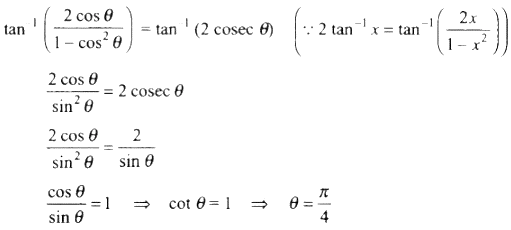10. Show that cos (2 tan-1 1/7) = sin (4 tan-1 1/3).

Solution:

Taking L.H.S, we have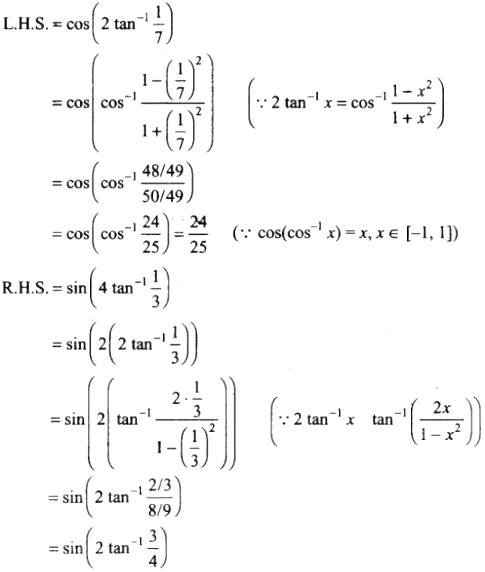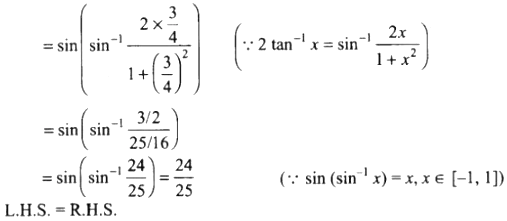Thus, L.H.S = R.H.S

– Hence proved

11. Solve the equation cos (tan-1 x) = sin (cot-1 3/4).

Solution:

Given equation, cos (tan-1 x) = sin (cot-1 3/4)

Taking L.H.S,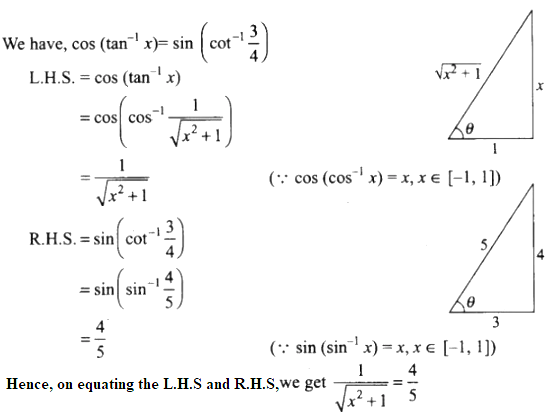On squaring on both sides,

16(x2 + 1) = 25

16x2 + 16 = 25

16x2 = 9

x2 = 9/16

Therefore, x = ± 3/4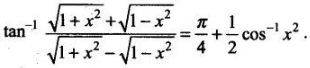12. Prove that

Solution: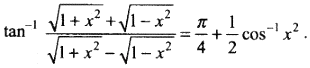Taking L.H.S,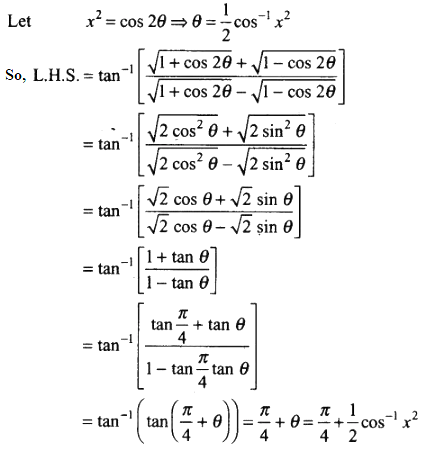= R.H.S

– Hence Proved

13. Find the simplified form of cos-1 [3/5 cos x + 4/5 sin x], x ∈ [-3π/4, π/4].

Solution:

We have,

cos-1 [3/5 cos x + 4/5 sin x], x ∈ [-3π/4, π/4]

Now, let cos α = 3/5

So, sin α = 4/5 and tan α = 4/3

cos-1 [3/5 cos x + 4/5 sin x]

⇒ cos-1 [3/5 cos x + 4/5 sin x] = cos-1 [cos α cos x + sin α sin x]

= cos-1 [cos (α – x)]

= α – x

= tan-1 4/3 – x

14. Prove that sin-1 8/17 + sin-1 3/5 = sin-1 77/85

Solution:

Taking the L.H.S,

= sin-1 8/17 + sin-1 3/5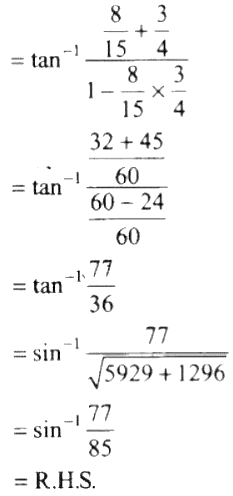= tan-1 8/15 + tan-1 3/4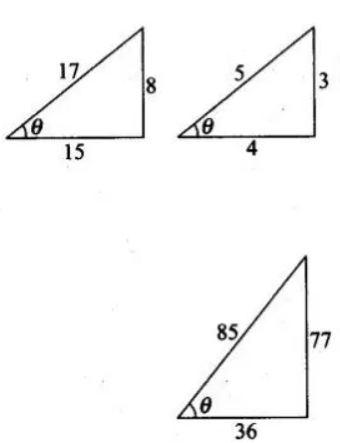– Hence proved

15. Show that sin-1 5/13 + cos-1 3/5 = tan-1 63/16

Solution:

Here, sin-1 5/13 = tan-1 5/12

And,

cos-1 3/5 = tan-1 4/3

Taking the L.H.S, we have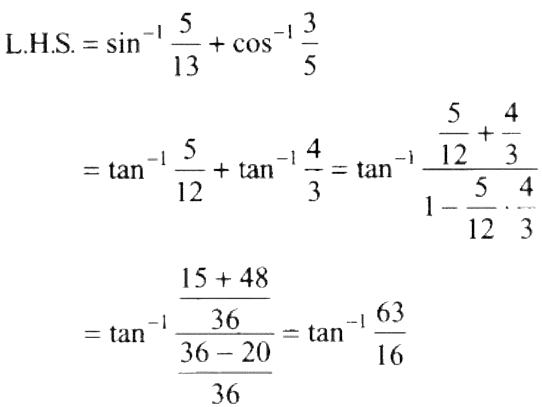Thus, L.H.S = R.H.S

– Hence Proved

16. Prove that tan-1 1/4 + tan-1 2/9 = sin-1 1/√5

Solution:

Taking the LHS,

tan-1 1/4 + tan-1 2/9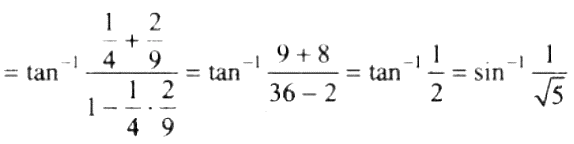= RHS

– Hence Proved

17. Find the value of 4 tan-1 1/5 – tan-1 1/239

Solution:

4 tan-1 1/5 – tan-1 1/239

= 2 (tan-1 1/5) – tan-1 1/239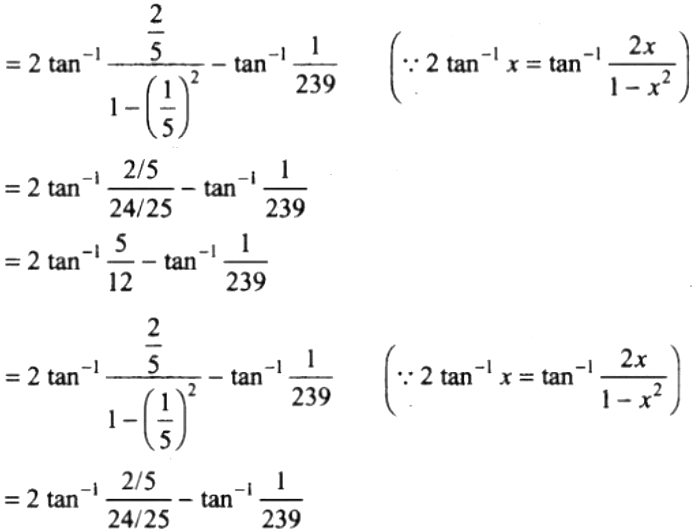= 2 tan-1 5/12 – tan-1 1/239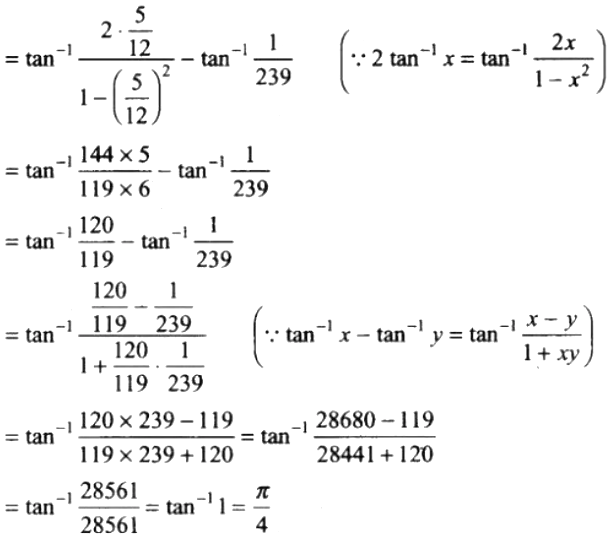Thus,

4 tan-1 1/5 – tan-1 1/239 = π/4

18. Show that tan-1 (1/2 sin-1 3/4) = (4 – √7)/ 3 and justify why the other value (4 + √7)/ 3 is ignored.

Solution:

We have, tan-1 (1/2 sin-1 3/4)

Let ½ sin-1 ¾ = θ ⇒ sin-1 ¾ = 2θ ⇒ sin 2θ = ¾

2 tan θ/ 1 + tan2 θ = ¾

3 tan2 θ – 8 tan θ + 3 = 0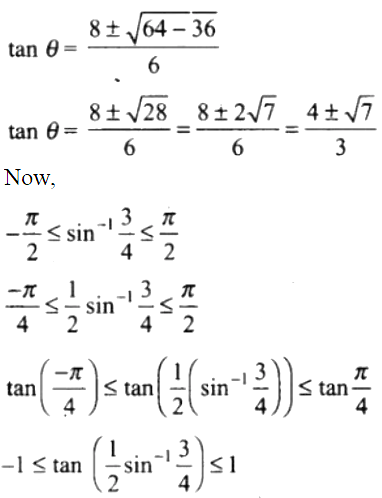Hence,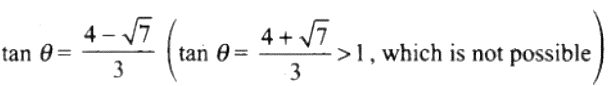19. If a1, a2, a3, …., an is an arithmetic progression with common difference d, then evaluate the following expression.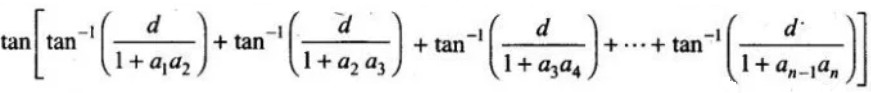Solution:

As a1, a2, a3, …., an is an arithmetic progression with common difference d.

d = a2 – a1 = a3 – a2 = a4 – a3 = …… = an – an-1

So,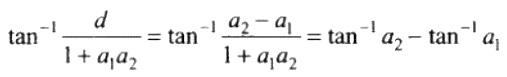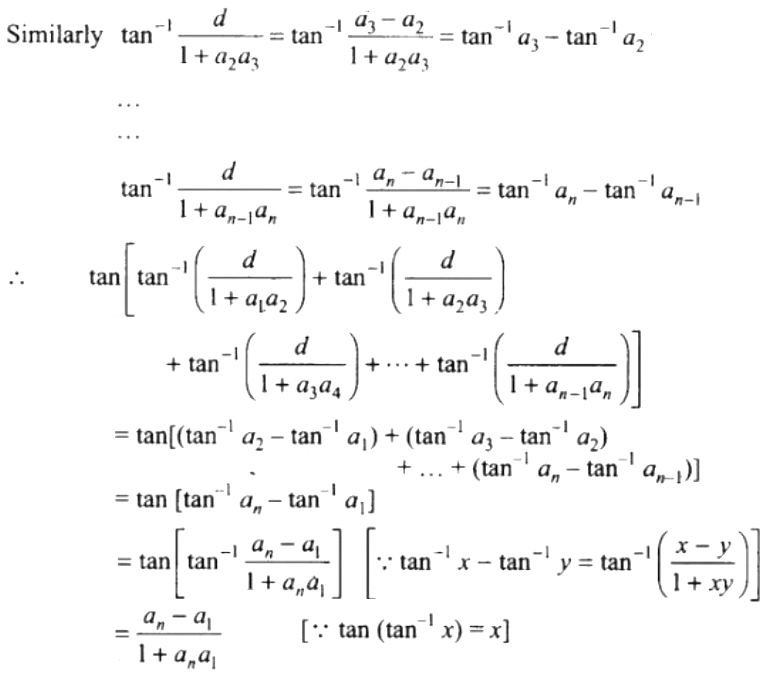20. Which of the following is the principal value branch of cos-1 x?

(a) [-π/2, π/2] (b) (0, π) (c) [0. π] (d) [0, π] – {π/2}

Solution:

(c) [0. π]

As we know that the principal value branch cos-1 x is [0, π].

21. Which of the following is the principal value branch of cosec-1 x?

(a) (-π/2, π/2) (b) [0, π] – {π/2} (c) [-π/2, π/2] (d) [-π/2, π/2] – {0}

Solution:

(d) [-π/2, π/2] – {0}

As the principal branch of cosec-1 x is [-π/2, π/2] – {0}.

22. If 3 tan-1 x + cot-1 x = π, then x equals

(a) 0 (b) 1 (c) -1 (d) ½

Solution:

(b) 1

Given, 3 tan-1 x + cot-1 x = π

2 tan-1 x + tan-1 x + cot-1 x = π

2 tan-1 x + π/2 = π (As tan-1 + cot-1 = π/2)

2 tan-1 x = π/2

tan-1 x = π/4

x = 1

23. The value of sin-1 cos 33π/5 is

(a) 3π/5 (b) -7π/5 (c) π/10 (d) -π/10

Solution:

(d) -π/10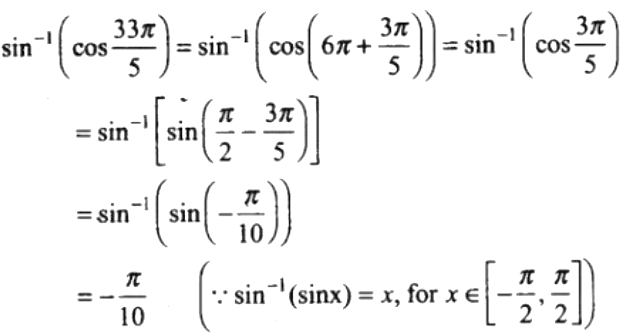24. The domain of the function cos-1 (2x – 1) is

(a) [0, 1] (b) [-1, 1] (c) [-1, 1] (d) [0, π]

Solution:

(a) [0, 1]

Since, cos-1 x is defined for x ∈ [-1, 1]

So, f(x) = cos-1 (2x – 1) is defined if

-1 ≤ 2x – 1 ≤ 1

0 ≤ 2x ≤ 2

Hence,

0 ≤ x ≤ 1

25. The domain of the function by f(x) = sin-1 √(x – 1) is

(a) [1, 2] (b) [-1, 1] (c) [0, 1] (d) none of these

Solution:

(a) [1, 2]

We know that, sin-1 x is defined for x ∈ [-1, 1]

So, f(x) = sin-1 √(x – 1) is defined if

0 ≤ √(x – 1) ≤ 1

0 ≤ x – 1 ≤ 1

1 ≤ x ≤ 2

Hence,

x ∈ [1, 2]

26. If cos (sin-1 2/5 + cos-1 x) = 0, then x is equal to

(a) 1/5 (b) 2/5 (c) 0 (d) 1

Solution:

(b) 2/5

Given,

cos (sin-1 2/5 + cos-1 x) = 0

So, this can be rewritten as

sin-1 2/5 + cos-1 x = cos-1 0

sin-1 2/5 + cos-1 x = π/2

cos-1 x = π/2 – sin-1 2/5

cos-1 x = cos-1 2/5 [Since, cos-1 x + sin-1 x = π/2]

Hence,

x = 2/5

27. The value of sin (2 tan-1(0.75)) is equal to

(a) 0.75 (b) 1.5 (c) 0.96 (d) sin 1.5

Solution:

(c) 0.96

We have, sin (2 tan-1(0.75))